Research papers and code for "Olivier Fercoq":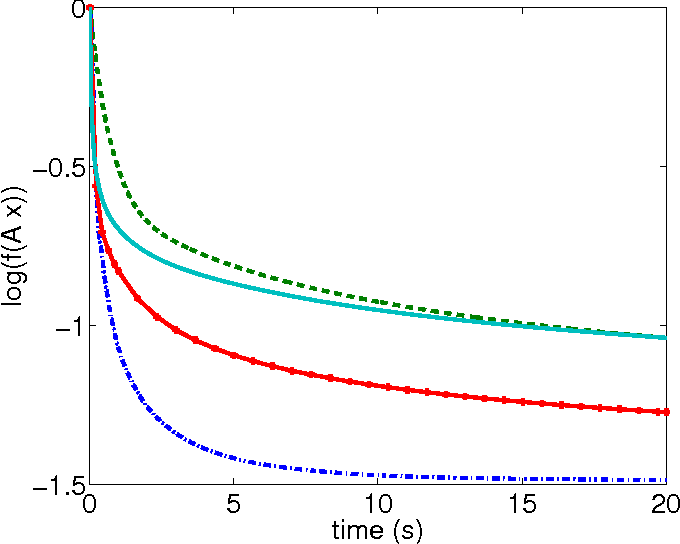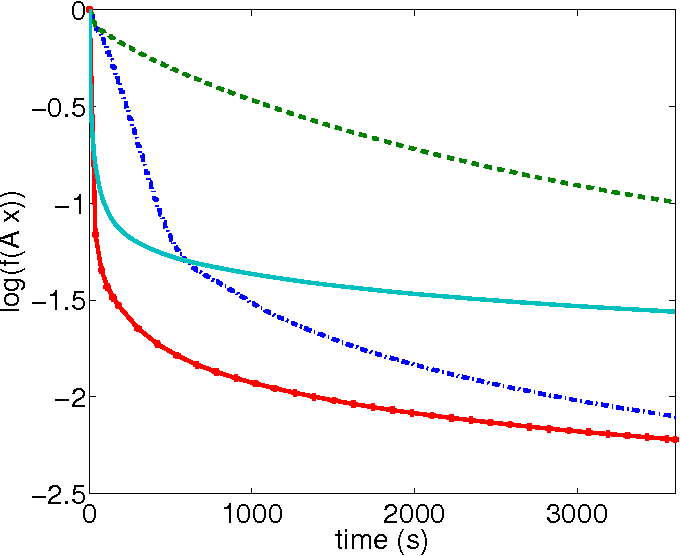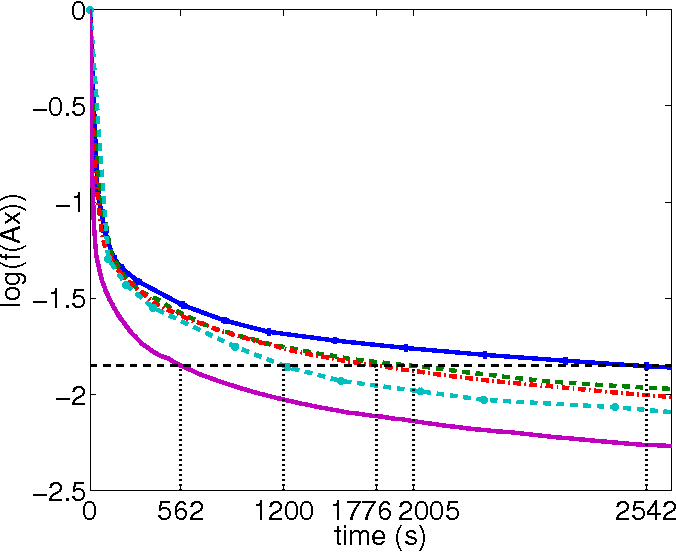We design a randomised parallel version of Adaboost based on previous studies on parallel coordinate descent. The algorithm uses the fact that the logarithm of the exponential loss is a function with coordinate-wise Lipschitz continuous gradient, in order to define the step lengths. We provide the proof of convergence for this randomised Adaboost algorithm and a theoretical parallelisation speedup factor. We finally provide numerical examples on learning problems of various sizes that show that the algorithm is competitive with concurrent approaches, especially for large scale problems.

* 7 pages, 3 figures, extended version of the paper presented to ICMLA'13
Click to Read Paper and Get Code
We propose a new stochastic coordinate descent method for minimizing the sum of convex functions each of which depends on a small number of coordinates only. Our method (APPROX) is simultaneously Accelerated, Parallel and PROXimal; this is the first time such a method is proposed. In the special case when the number of processors is equal to the number of coordinates, the method converges at the rate $2\bar{\omega}\bar{L} R^2/(k+1)^2$, where $k$ is the iteration counter, $\bar{\omega}$ is an average degree of separability of the loss function, $\bar{L}$ is the average of Lipschitz constants associated with the coordinates and individual functions in the sum, and $R$ is the distance of the initial point from the minimizer. We show that the method can be implemented without the need to perform full-dimensional vector operations, which is the major bottleneck of existing accelerated coordinate descent methods. The fact that the method depends on the average degree of separability, and not on the maximum degree of separability, can be attributed to the use of new safe large stepsizes, leading to improved expected separable overapproximation (ESO). These are of independent interest and can be utilized in all existing parallel stochastic coordinate descent algorithms based on the concept of ESO.

* 25 pages, 2 algorithms, 6 tables, 3 figures
Click to Read Paper and Get Code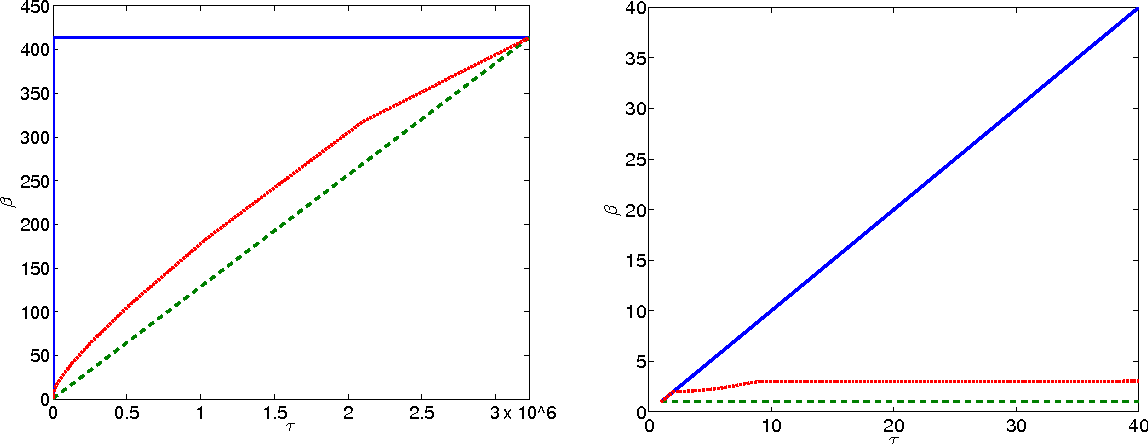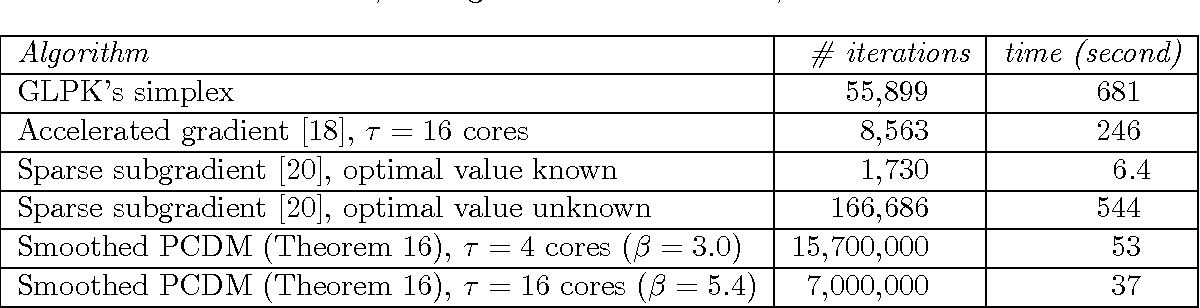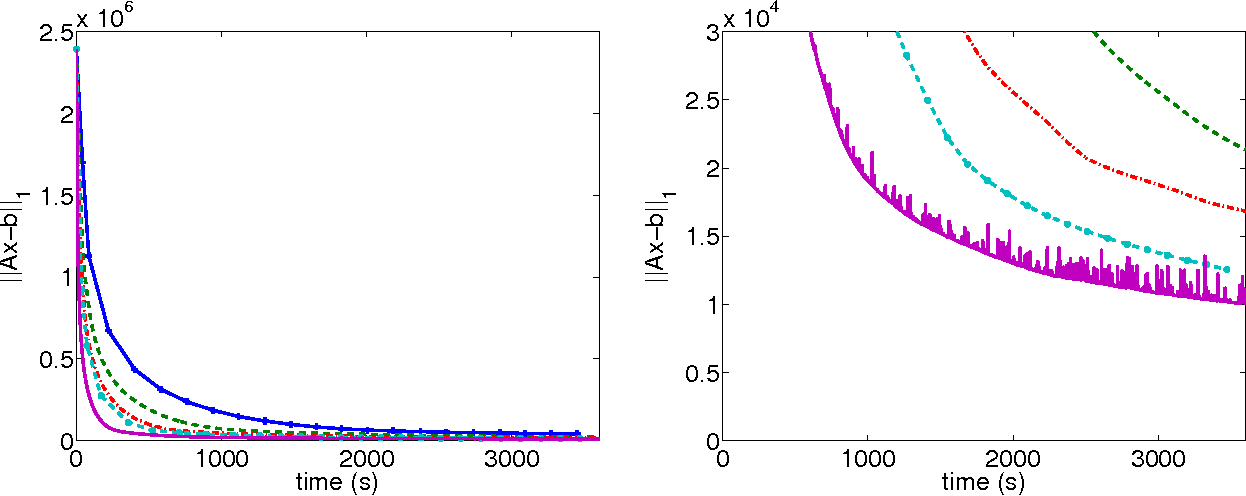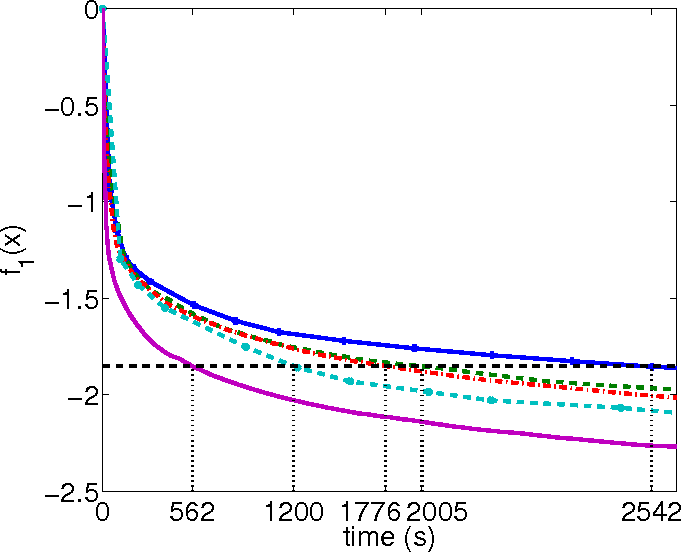We study the performance of a family of randomized parallel coordinate descent methods for minimizing the sum of a nonsmooth and separable convex functions. The problem class includes as a special case L1-regularized L1 regression and the minimization of the exponential loss ("AdaBoost problem"). We assume the input data defining the loss function is contained in a sparse $m\times n$ matrix $A$ with at most $\omega$ nonzeros in each row. Our methods need $O(n \beta/\tau)$ iterations to find an approximate solution with high probability, where $\tau$ is the number of processors and $\beta = 1 + (\omega-1)(\tau-1)/(n-1)$ for the fastest variant. The notation hides dependence on quantities such as the required accuracy and confidence levels and the distance of the starting iterate from an optimal point. Since $\beta/\tau$ is a decreasing function of $\tau$, the method needs fewer iterations when more processors are used. Certain variants of our algorithms perform on average only $O(\nnz(A)/n)$ arithmetic operations during a single iteration per processor and, because $\beta$ decreases when $\omega$ does, fewer iterations are needed for sparser problems.

* 39 pages, 1 algorithm, 3 figures, 2 tables
Click to Read Paper and Get Code
Screening rules allow to early discard irrelevant variables from the optimization in Lasso problems, or its derivatives, making solvers faster. In this paper, we propose new versions of the so-called $\textit{safe rules}$ for the Lasso. Based on duality gap considerations, our new rules create safe test regions whose diameters converge to zero, provided that one relies on a converging solver. This property helps screening out more variables, for a wider range of regularization parameter values. In addition to faster convergence, we prove that we correctly identify the active sets (supports) of the solutions in finite time. While our proposed strategy can cope with any solver, its performance is demonstrated using a coordinate descent algorithm particularly adapted to machine learning use cases. Significant computing time reductions are obtained with respect to previous safe rules.

* erratum to ICML 2015, "The authors would like to thanks Jalal Fadili and Jingwei Liang for helping clarifying some misleading statements on the equicorrelation set"
Click to Read Paper and Get Code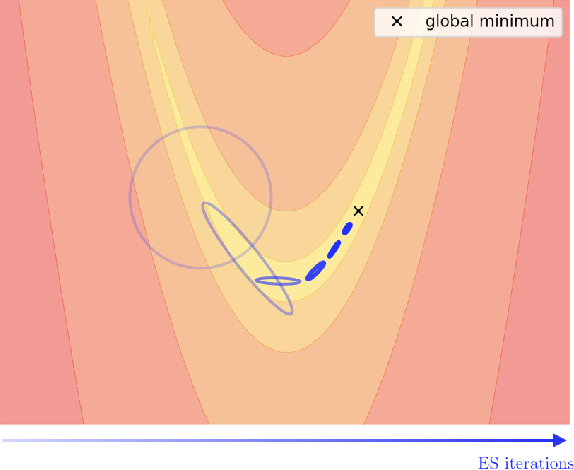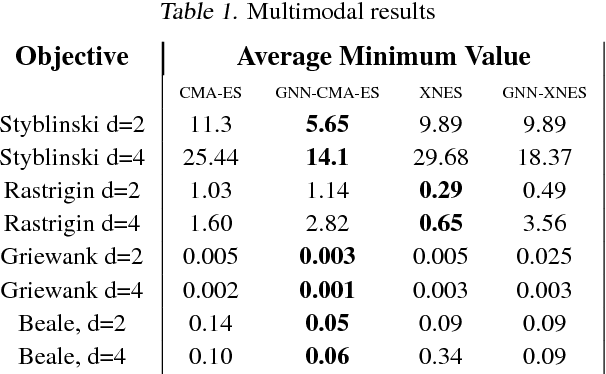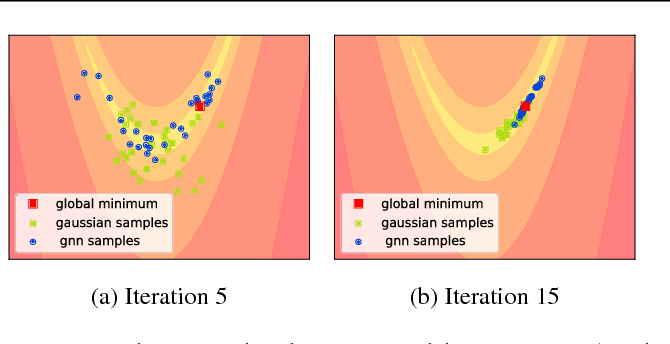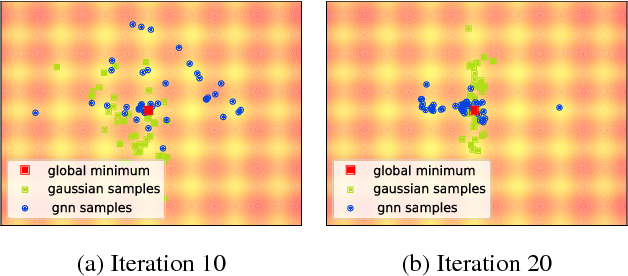Evolutionary Strategies (ES) are a popular family of black-box zeroth-order optimization algorithms which rely on search distributions to efficiently optimize a large variety of objective functions. This paper investigates the potential benefits of using highly flexible search distributions in classical ES algorithms, in contrast to standard ones (typically Gaussians). We model such distributions with Generative Neural Networks (GNNs) and introduce a new training algorithm that leverages their expressiveness to accelerate the ES procedure. We show that this tailored algorithm can readily incorporate existing ES algorithms, and outperforms the state-of-the-art on diverse objective functions.

Click to Read Paper and Get Code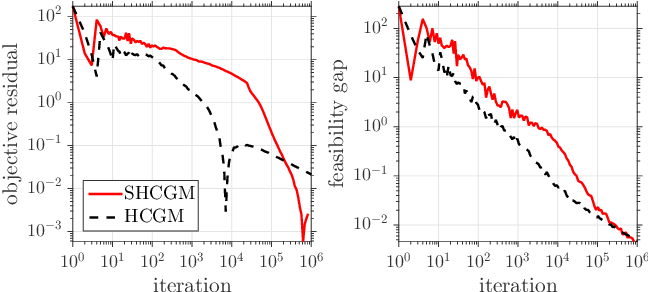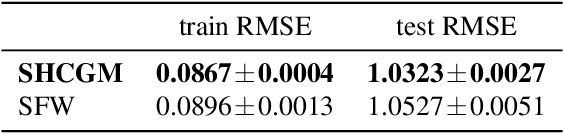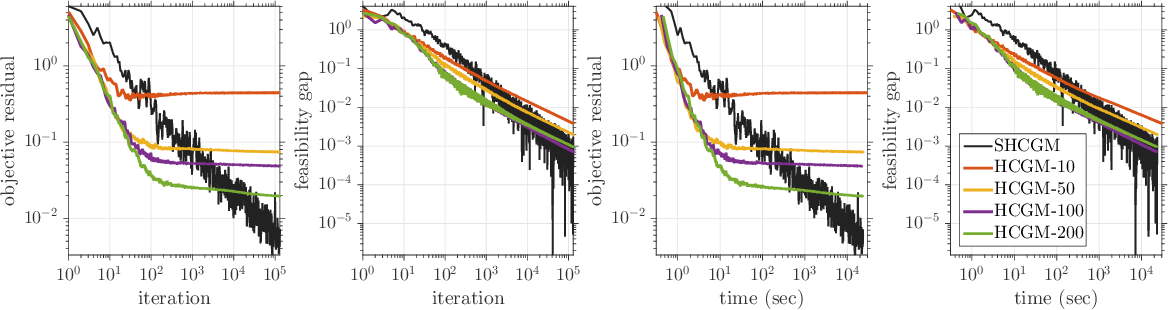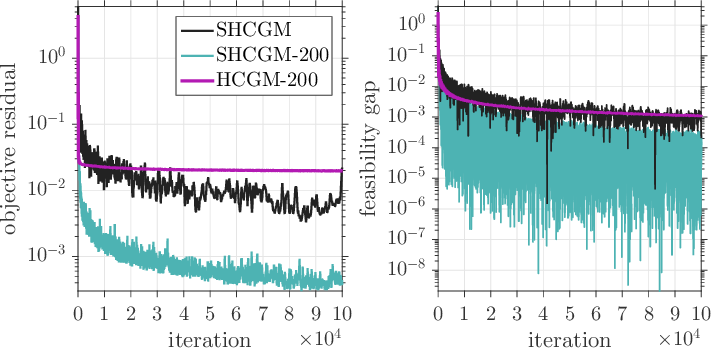In this paper, we propose the first practical algorithm to minimize stochastic composite optimization problems over compact convex sets. This template allows for affine constraints and therefore covers stochastic semidefinite programs (SDPs), which are vastly applicable in both machine learning and statistics. In this setup, stochastic algorithms with convergence guarantees are either not known or not tractable. We tackle this general problem and propose a convergent, easy to implement and tractable algorithm. We prove $\mathcal{O}(k^{-1/3})$ convergence rate in expectation on the objective residual and $\mathcal{O}(k^{-5/12})$ in expectation on the feasibility gap. These rates are achieved without increasing the batchsize, which can contain a single sample. We present extensive empirical evidence demonstrating the superiority of our algorithm on a broad range of applications including optimization of stochastic SDPs.

Click to Read Paper and Get Code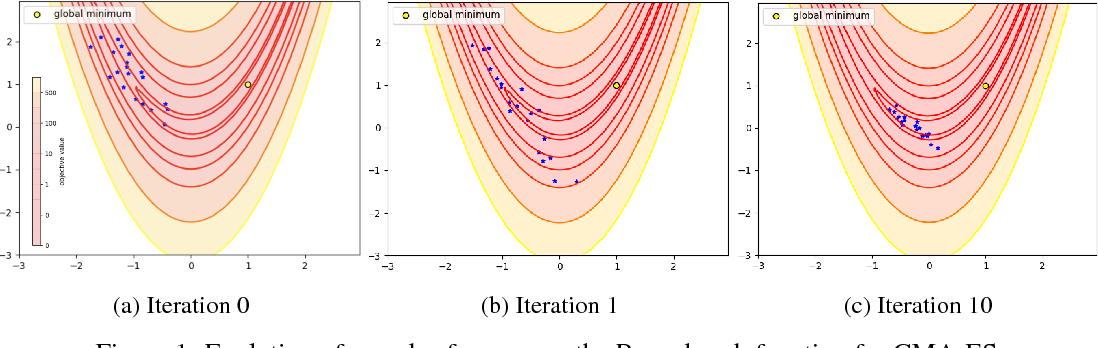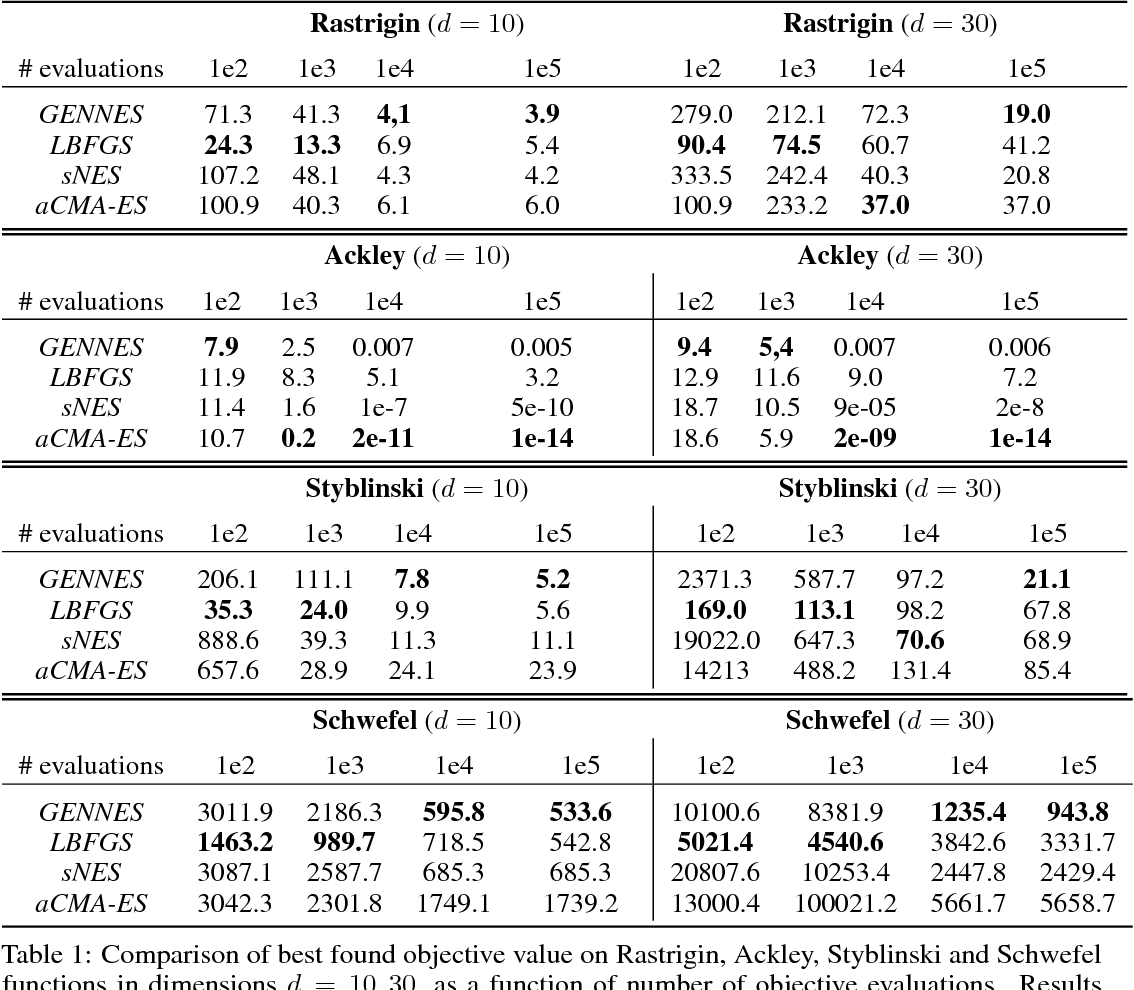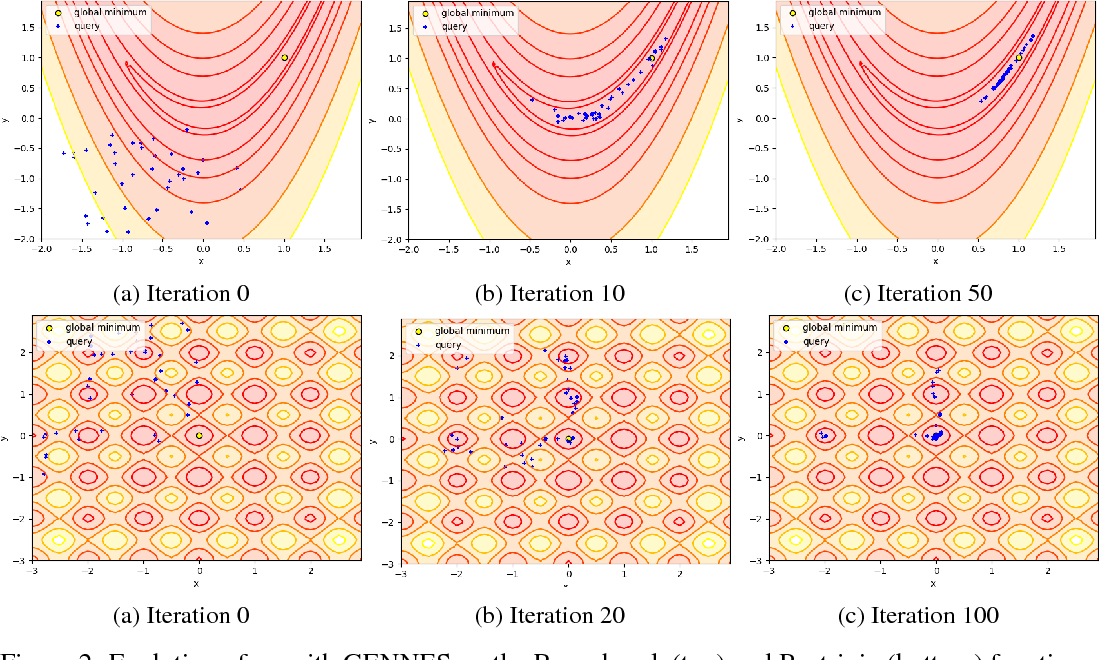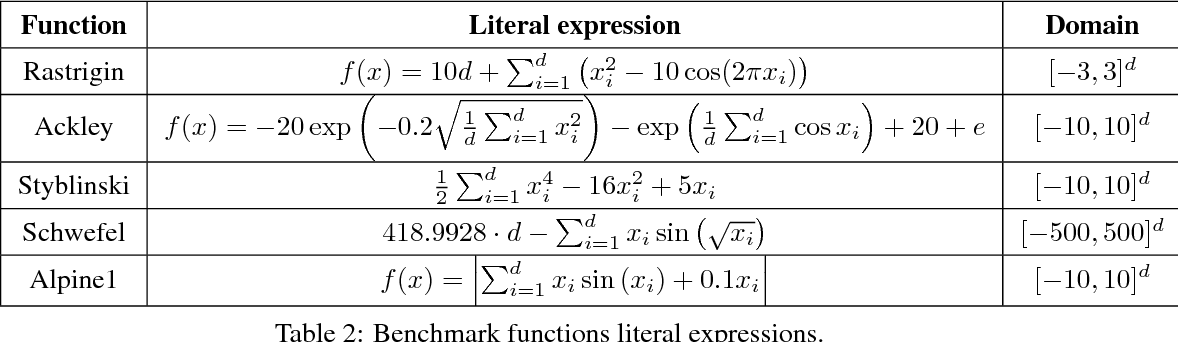The aim of global optimization is to find the global optimum of arbitrary classes of functions, possibly highly multimodal ones. In this paper we focus on the subproblem of global optimization for differentiable functions and we propose an Evolutionary Search-inspired solution where we model point search distributions via Generative Neural Networks. This approach enables us to model diverse and complex search distributions based on which we can efficiently explore complicated objective landscapes. In our experiments we show the practical superiority of our algorithm versus classical Evolutionary Search and gradient-based solutions on a benchmark set of multimodal functions, and demonstrate how it can be used to accelerate Bayesian Optimization with Gaussian Processes.

Click to Read Paper and Get Code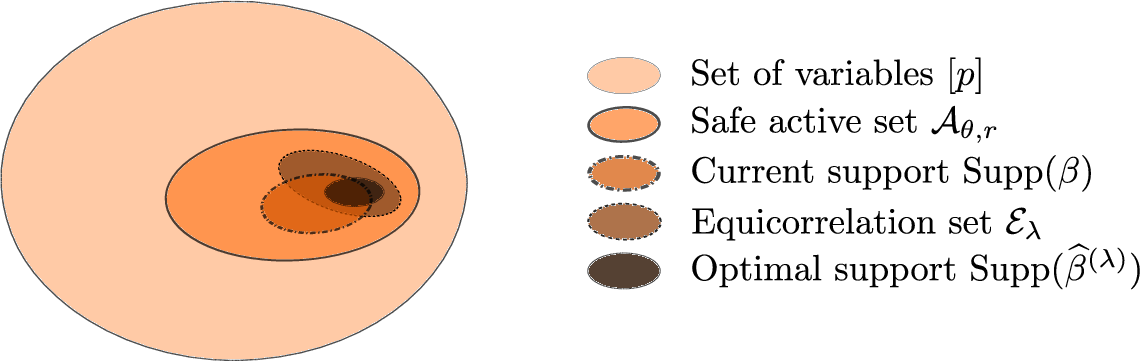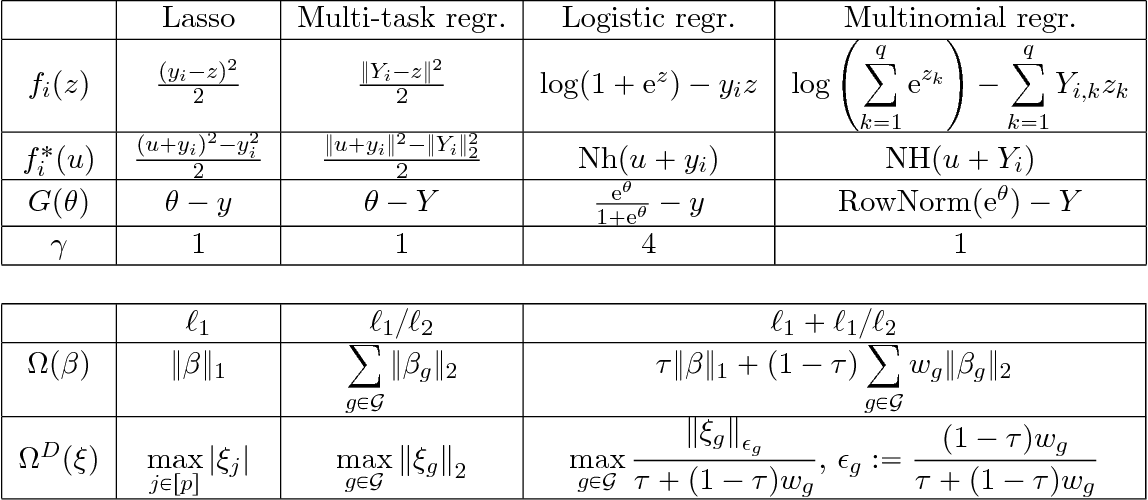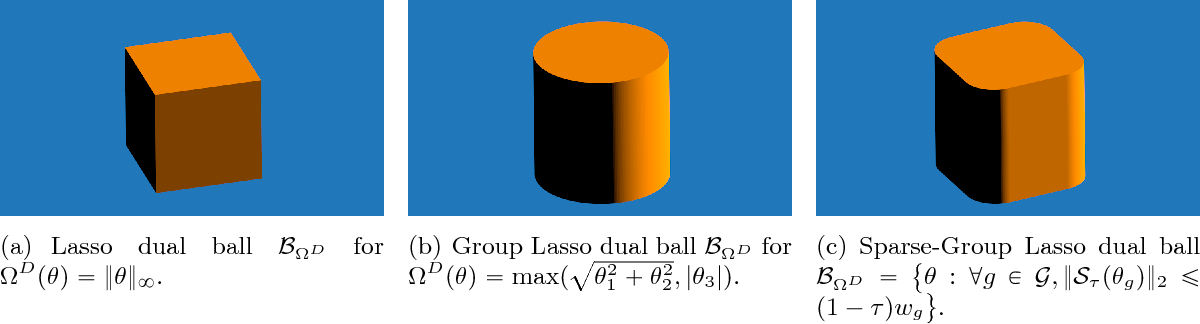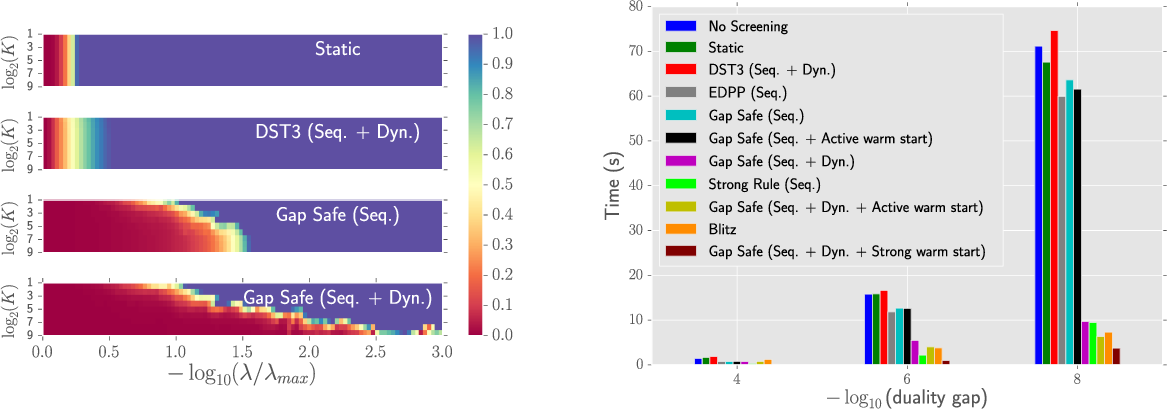In high dimensional regression settings, sparsity enforcing penalties have proved useful to regularize the data-fitting term. A recently introduced technique called screening rules propose to ignore some variables in the optimization leveraging the expected sparsity of the solutions and consequently leading to faster solvers. When the procedure is guaranteed not to discard variables wrongly the rules are said to be safe. In this work, we propose a unifying framework for generalized linear models regularized with standard sparsity enforcing penalties such as $\ell_1$ or $\ell_1/\ell_2$ norms. Our technique allows to discard safely more variables than previously considered safe rules, particularly for low regularization parameters. Our proposed Gap Safe rules (so called because they rely on duality gap computation) can cope with any iterative solver but are particularly well suited to (block) coordinate descent methods. Applied to many standard learning tasks, Lasso, Sparse-Group Lasso, multi-task Lasso, binary and multinomial logistic regression, etc., we report significant speed-ups compared to previously proposed safe rules on all tested data sets.

Click to Read Paper and Get Code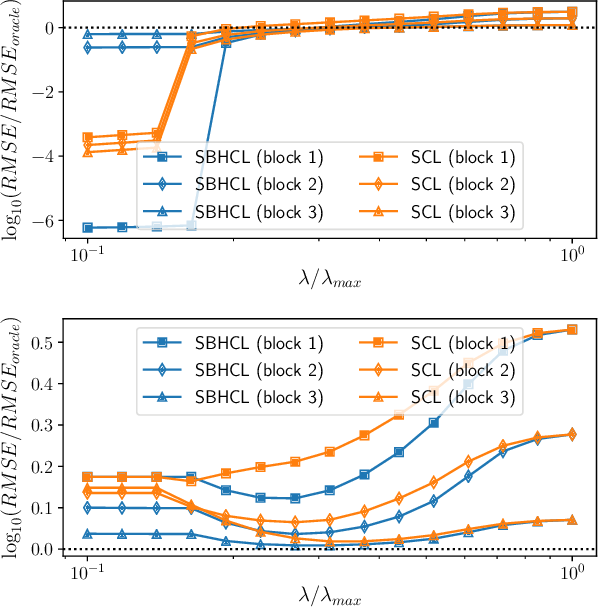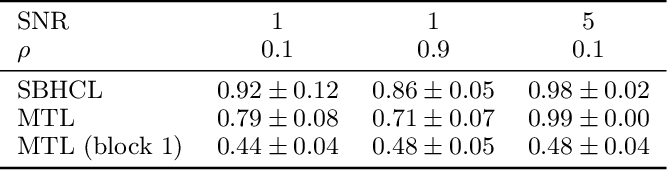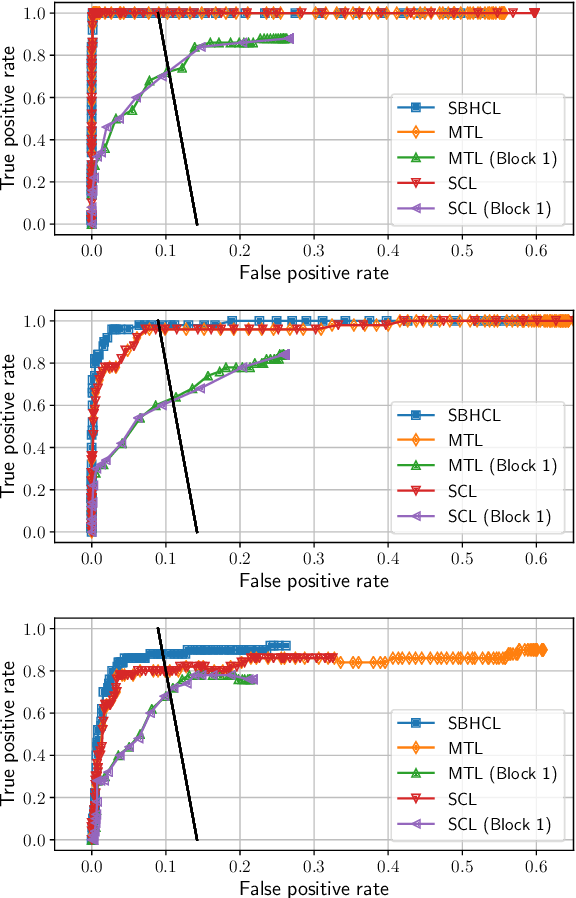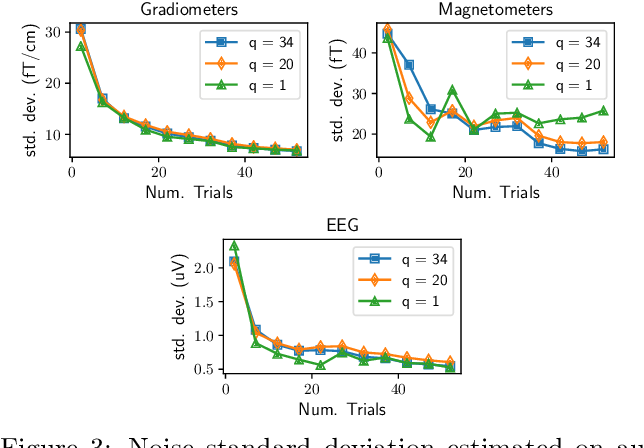In high dimension, it is customary to consider Lasso-type estimators to enforce sparsity. For standard Lasso theory to hold, the regularization parameter should be proportional to the noise level, yet the latter is generally unknown in practice. A possible remedy is to consider estimators, such as the Concomitant/Scaled Lasso, which jointly optimize over the regression coefficients as well as over the noise level, making the choice of the regularization independent of the noise level. However, when data from different sources are pooled to increase sample size, or when dealing with multimodal datasets, noise levels typically differ and new dedicated estimators are needed. In this work we provide new statistical and computational solutions to deal with such heteroscedastic regression models, with an emphasis on functional brain imaging with combined magneto- and electroencephalographic (M/EEG) signals. Adopting the formulation of Concomitant Lasso-type estimators, we propose a jointly convex formulation to estimate both the regression coefficients and the (square root of the) noise covariance. When our framework is instantiated to de-correlated noise, it leads to an efficient algorithm whose computational cost is not higher than for the Lasso and Concomitant Lasso, while addressing more complex noise structures. Numerical experiments demonstrate that our estimator yields improved prediction and support identification while correctly estimating the noise (square root) covariance. Results on multimodal neuroimaging problems with M/EEG data are also reported.

Click to Read Paper and Get Code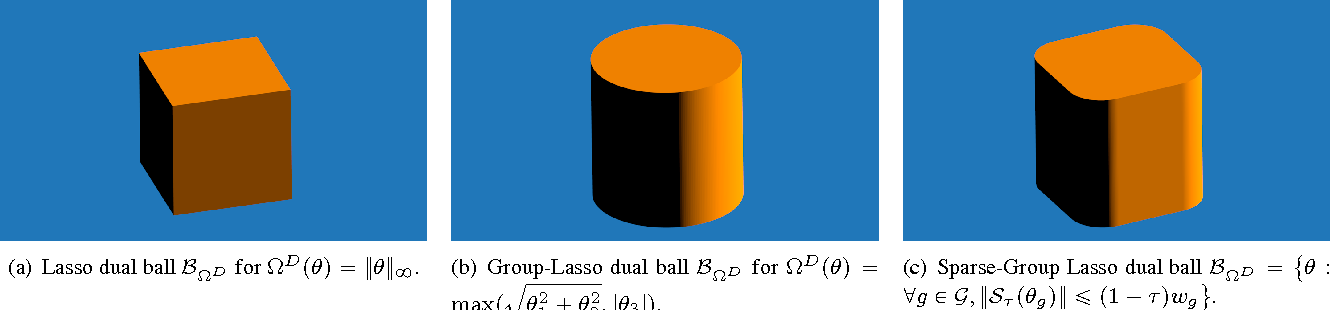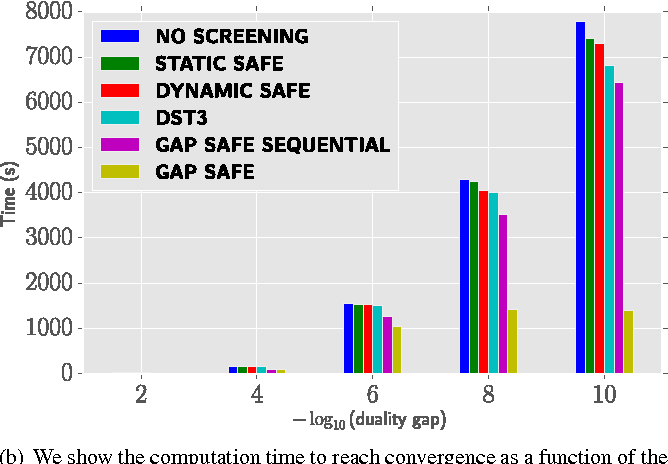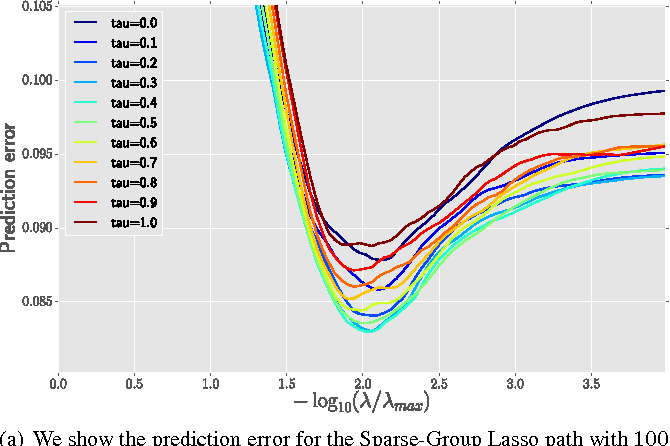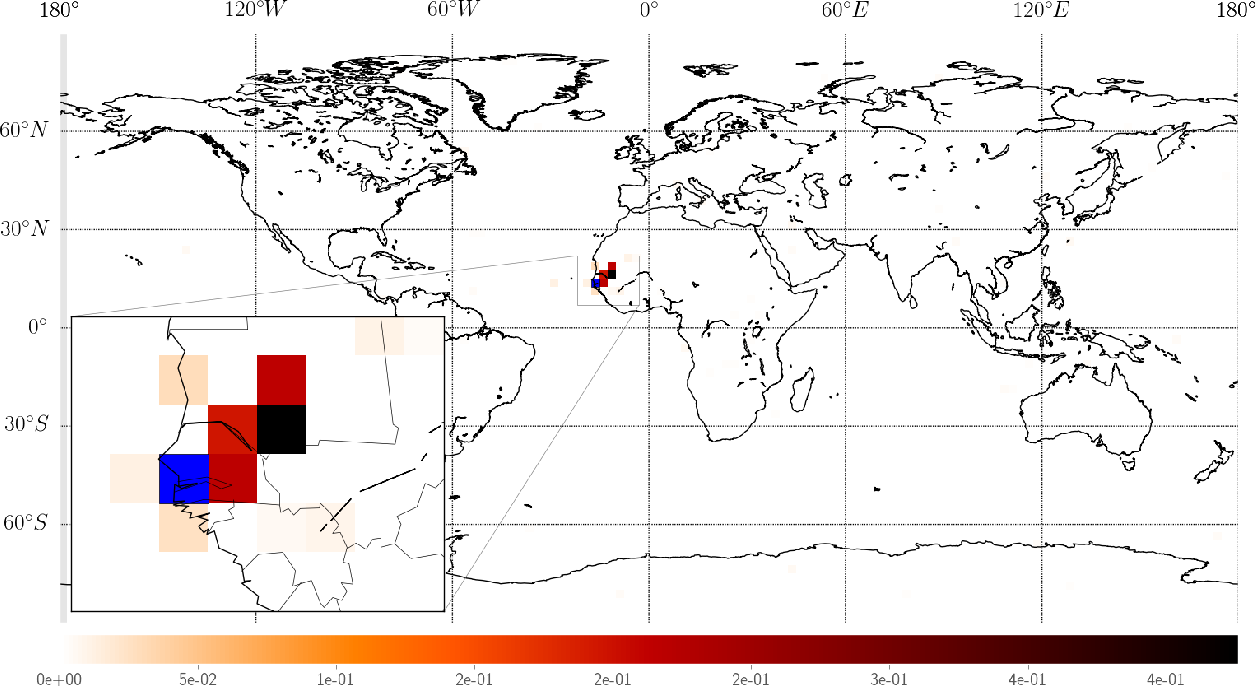In high dimensional settings, sparse structures are crucial for efficiency, either in term of memory, computation or performance. In some contexts, it is natural to handle more refined structures than pure sparsity, such as for instance group sparsity. Sparse-Group Lasso has recently been introduced in the context of linear regression to enforce sparsity both at the feature level and at the group level. We adapt to the case of Sparse-Group Lasso recent safe screening rules that discard early in the solver irrelevant features/groups. Such rules have led to important speed-ups for a wide range of iterative methods. Thanks to dual gap computations, we provide new safe screening rules for Sparse-Group Lasso and show significant gains in term of computing time for a coordinate descent implementation.

Click to Read Paper and Get Code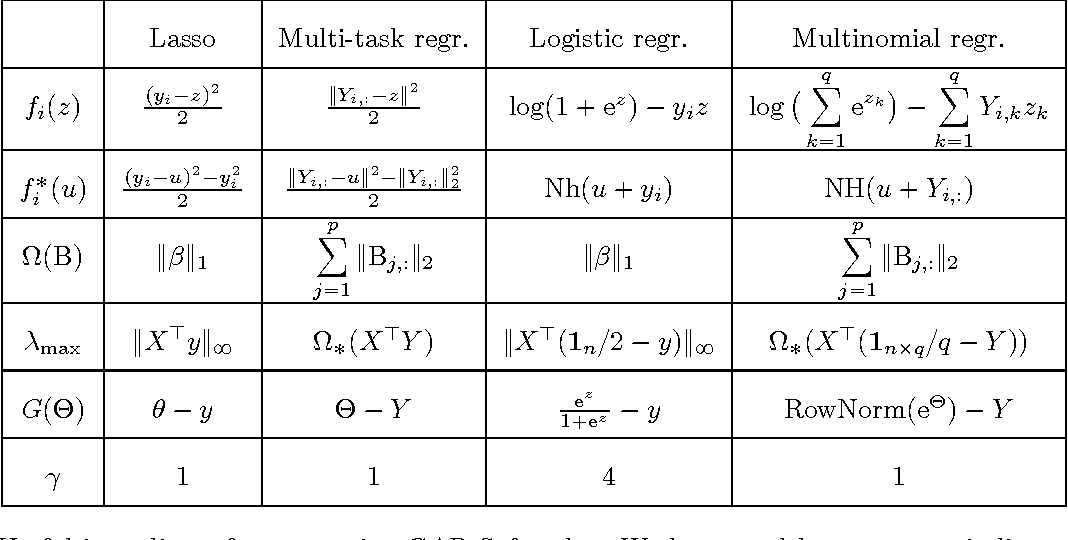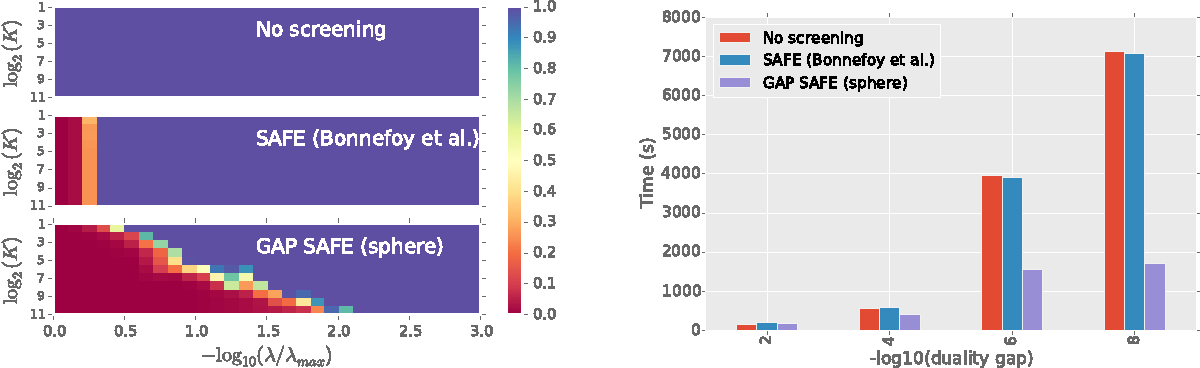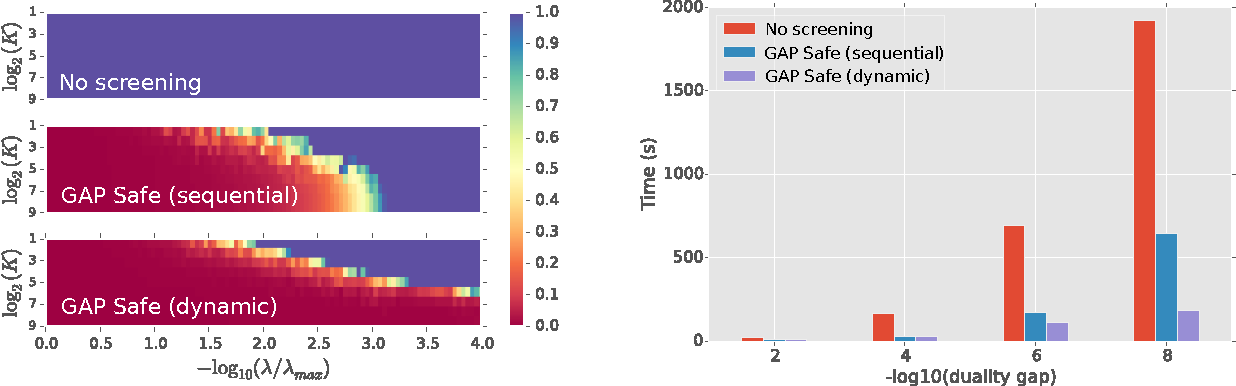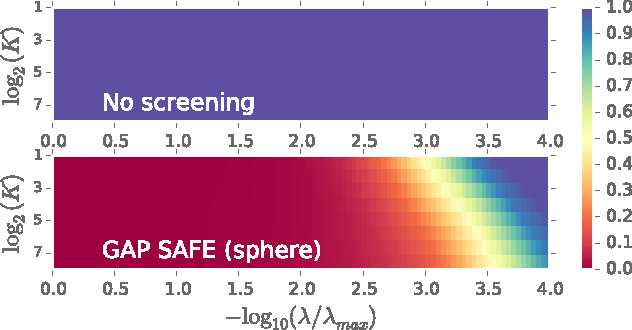High dimensional regression benefits from sparsity promoting regularizations. Screening rules leverage the known sparsity of the solution by ignoring some variables in the optimization, hence speeding up solvers. When the procedure is proven not to discard features wrongly the rules are said to be \emph{safe}. In this paper we derive new safe rules for generalized linear models regularized with $\ell_1$ and $\ell_1/\ell_2$ norms. The rules are based on duality gap computations and spherical safe regions whose diameters converge to zero. This allows to discard safely more variables, in particular for low regularization parameters. The GAP Safe rule can cope with any iterative solver and we illustrate its performance on coordinate descent for multi-task Lasso, binary and multinomial logistic regression, demonstrating significant speed ups on all tested datasets with respect to previous safe rules.

* in Proceedings of the 29-th Conference on Neural Information Processing Systems (NIPS), 2015
Click to Read Paper and Get Code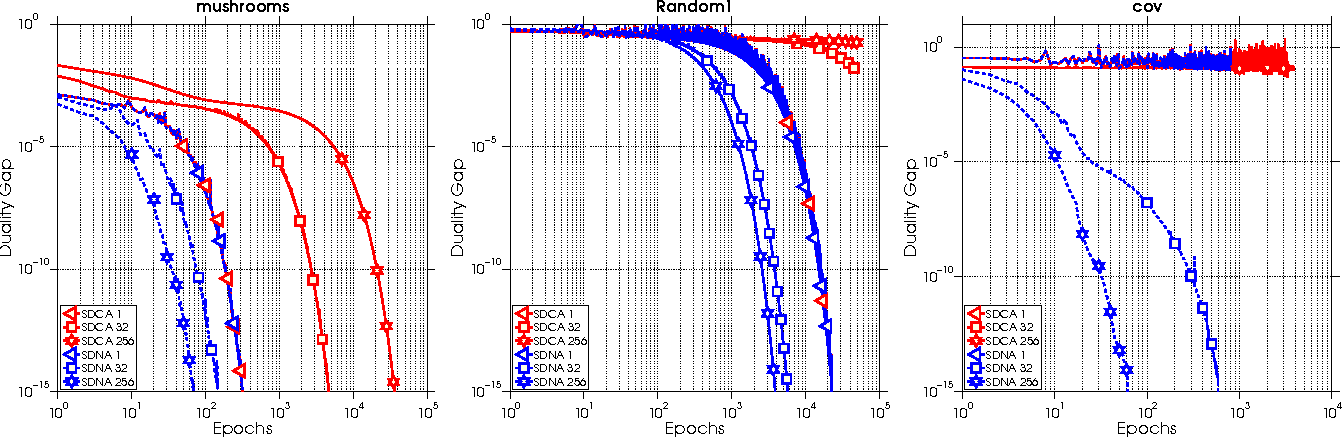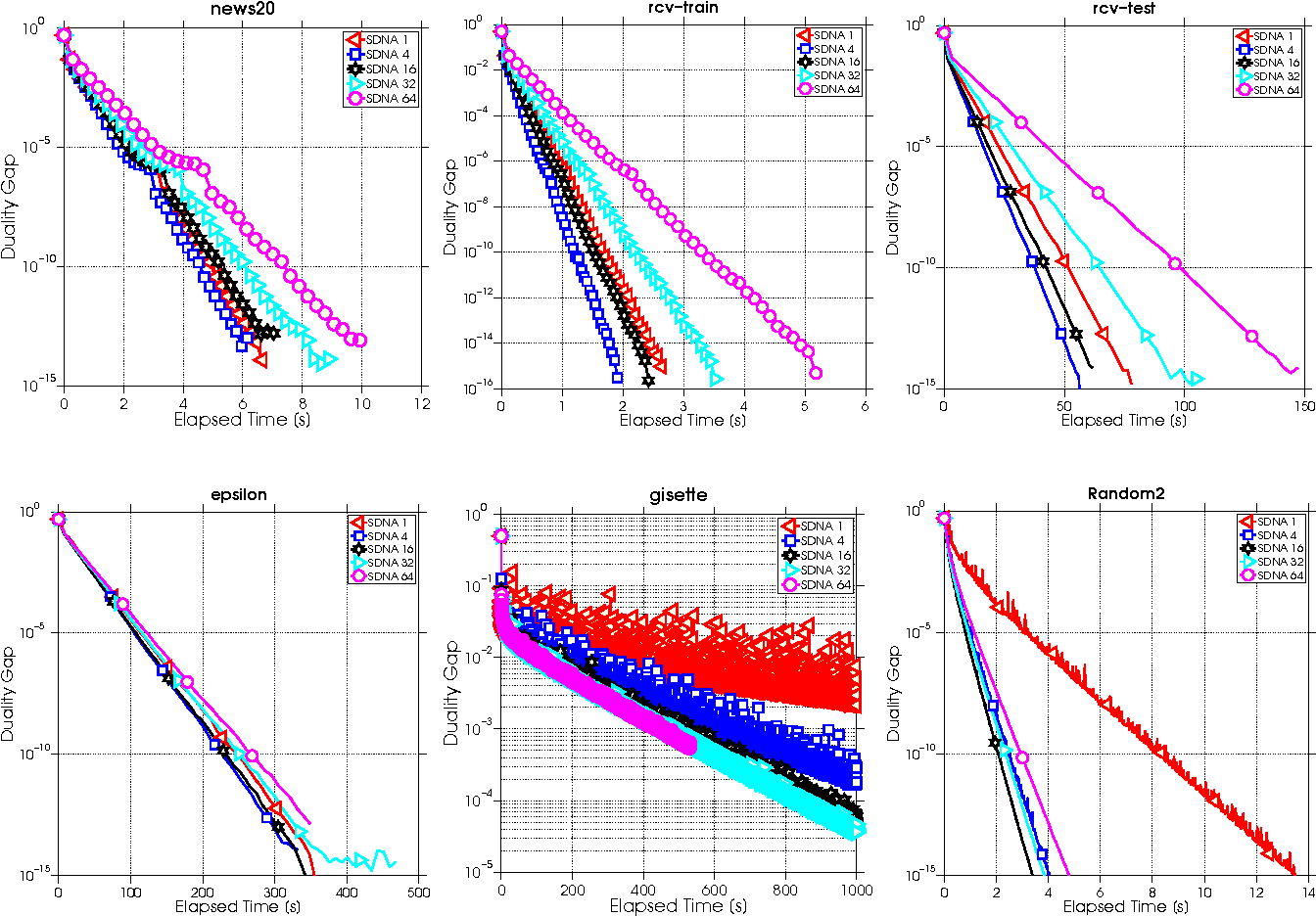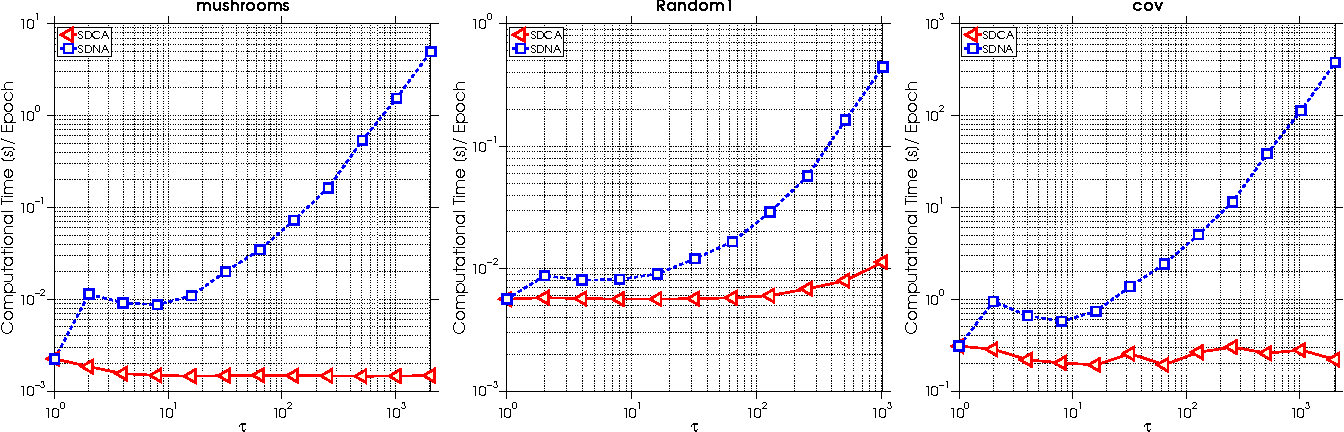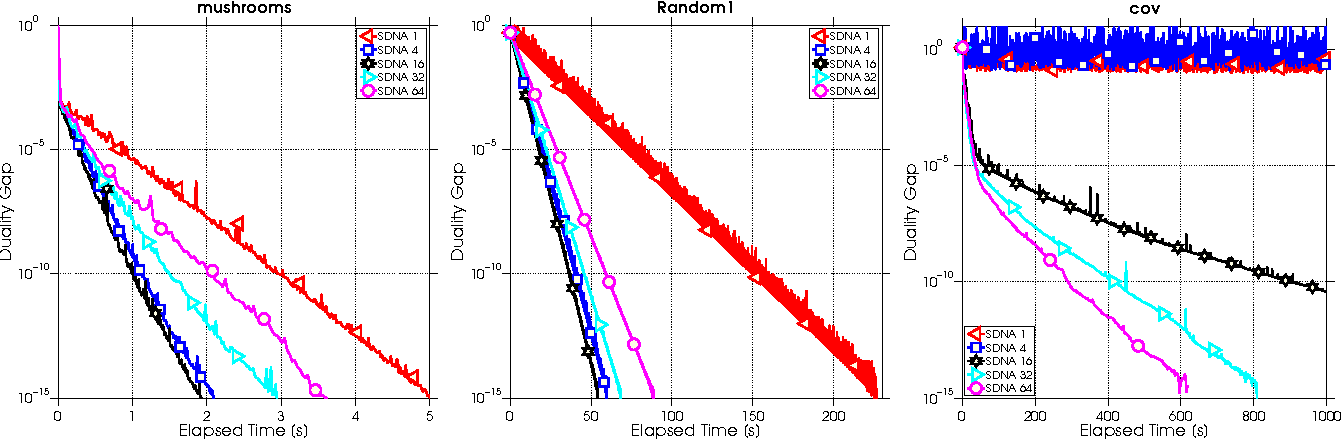We propose a new algorithm for minimizing regularized empirical loss: Stochastic Dual Newton Ascent (SDNA). Our method is dual in nature: in each iteration we update a random subset of the dual variables. However, unlike existing methods such as stochastic dual coordinate ascent, SDNA is capable of utilizing all curvature information contained in the examples, which leads to striking improvements in both theory and practice - sometimes by orders of magnitude. In the special case when an L2-regularizer is used in the primal, the dual problem is a concave quadratic maximization problem plus a separable term. In this regime, SDNA in each step solves a proximal subproblem involving a random principal submatrix of the Hessian of the quadratic function; whence the name of the method. If, in addition, the loss functions are quadratic, our method can be interpreted as a novel variant of the recently introduced Iterative Hessian Sketch.

Click to Read Paper and Get Code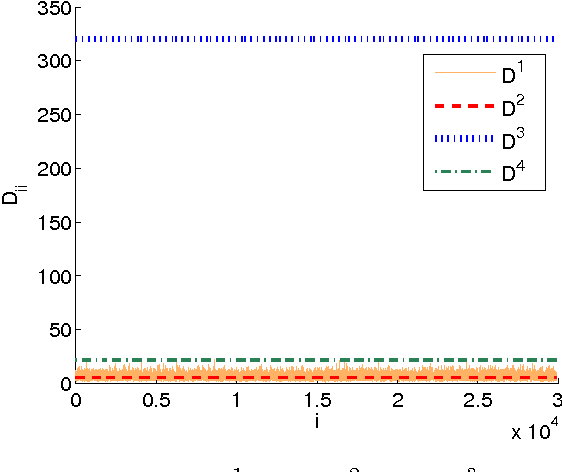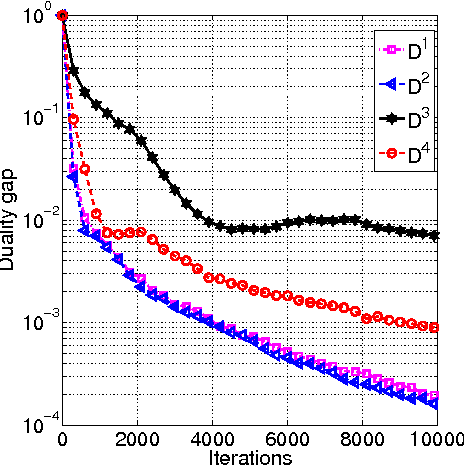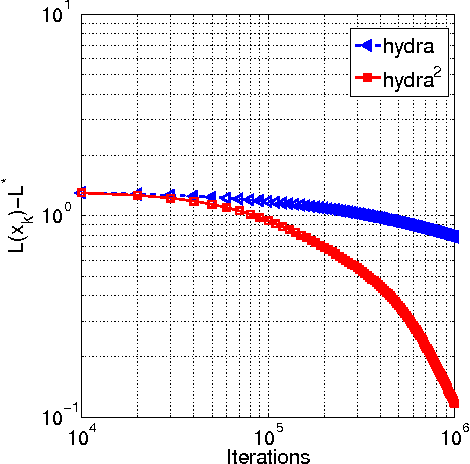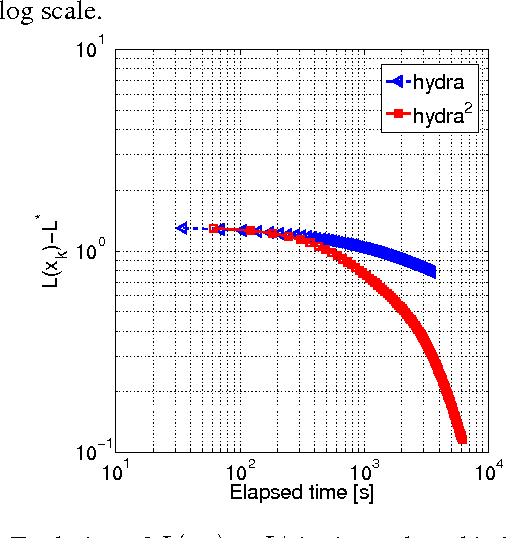We propose an efficient distributed randomized coordinate descent method for minimizing regularized non-strongly convex loss functions. The method attains the optimal $O(1/k^2)$ convergence rate, where $k$ is the iteration counter. The core of the work is the theoretical study of stepsize parameters. We have implemented the method on Archer - the largest supercomputer in the UK - and show that the method is capable of solving a (synthetic) LASSO optimization problem with 50 billion variables.

Click to Read Paper and Get Code
We propose a new randomized coordinate descent method for a convex optimization template with broad applications. Our analysis relies on a novel combination of four ideas applied to the primal-dual gap function: smoothing, acceleration, homotopy, and coordinate descent with non-uniform sampling. As a result, our method features the first convergence rate guarantees among the coordinate descent methods, that are the best-known under a variety of common structure assumptions on the template. We provide numerical evidence to support the theoretical results with a comparison to state-of-the-art algorithms.

* NIPS 2017
Click to Read Paper and Get Code
Popular machine learning estimators involve regularization parameters that can be challenging to tune, and standard strategies rely on grid search for this task. In this paper, we revisit the techniques of approximating the regularization path up to predefined tolerance $\epsilon$ in a unified framework and show that its complexity is $O(1/\sqrt[d]{\epsilon})$ for uniformly convex loss of order $d>0$ and $O(1/\sqrt{\epsilon})$ for Generalized Self-Concordant functions. This framework encompasses least-squares but also logistic regression (a case that as far as we know was not handled as precisely by previous works). We leverage our technique to provide refined bounds on the validation error as well as a practical algorithm for hyperparameter tuning. The later has global convergence guarantee when targeting a prescribed accuracy on the validation set. Last but not least, our approach helps relieving the practitioner from the (often neglected) task of selecting a stopping criterion when optimizing over the training set: our method automatically calibrates it based on the targeted accuracy on the validation set.

Click to Read Paper and Get Code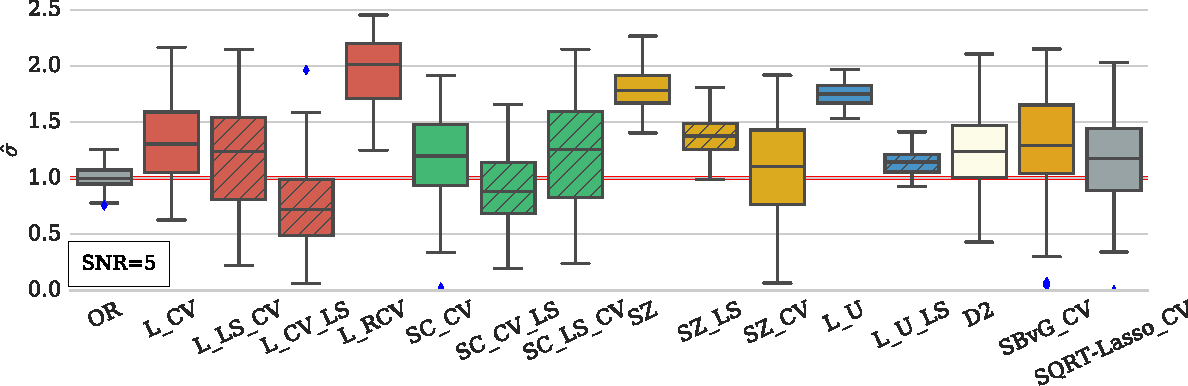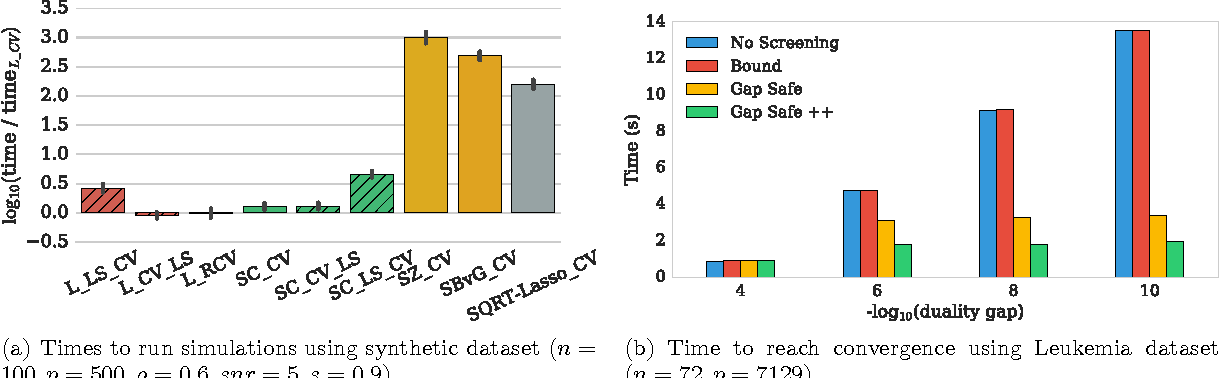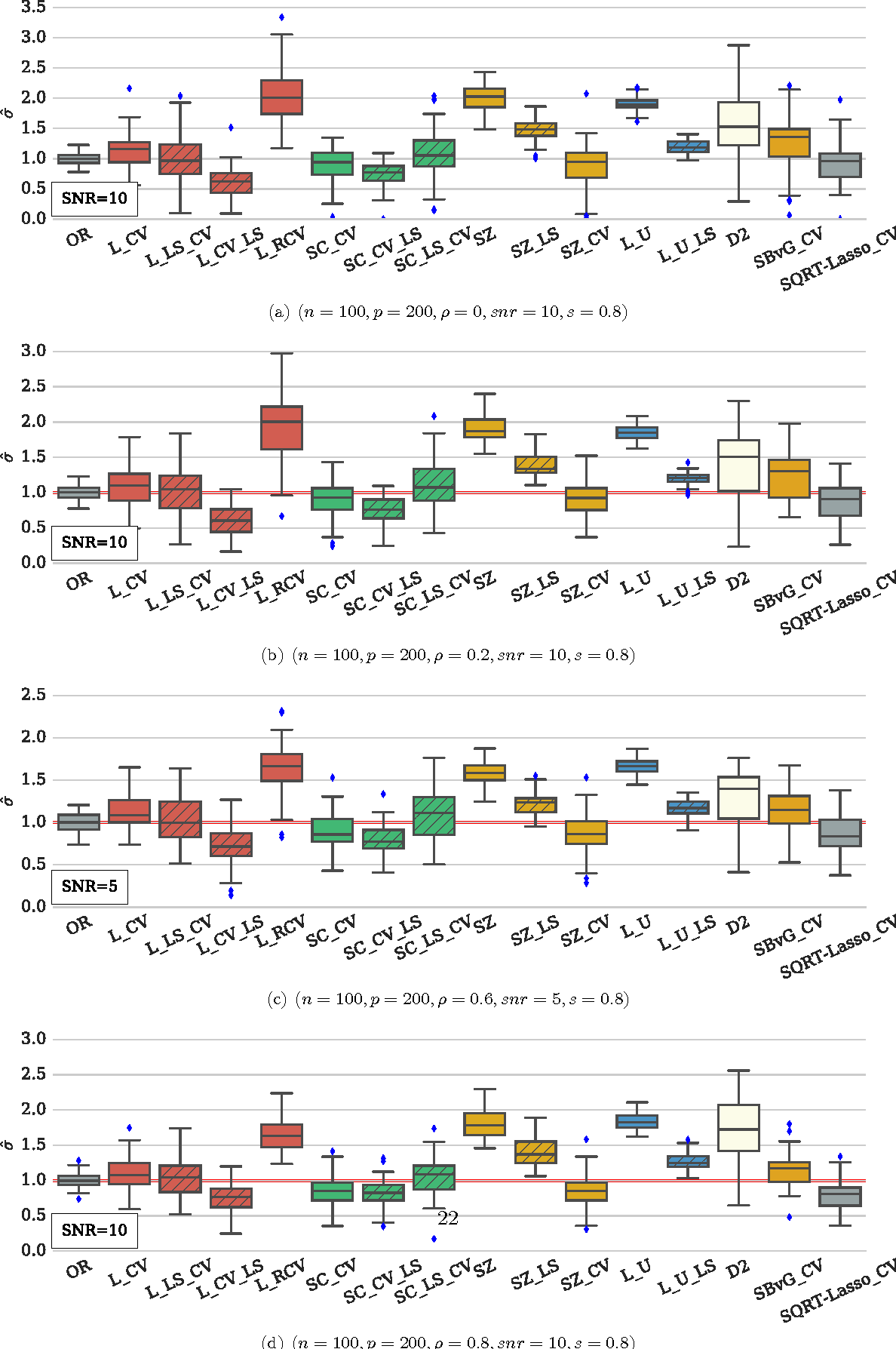In high dimensional settings, sparse structures are crucial for efficiency, both in term of memory, computation and performance. It is customary to consider $\ell_1$ penalty to enforce sparsity in such scenarios. Sparsity enforcing methods, the Lasso being a canonical example, are popular candidates to address high dimension. For efficiency, they rely on tuning a parameter trading data fitting versus sparsity. For the Lasso theory to hold this tuning parameter should be proportional to the noise level, yet the latter is often unknown in practice. A possible remedy is to jointly optimize over the regression parameter as well as over the noise level. This has been considered under several names in the literature: Scaled-Lasso, Square-root Lasso, Concomitant Lasso estimation for instance, and could be of interest for confidence sets or uncertainty quantification. In this work, after illustrating numerical difficulties for the Smoothed Concomitant Lasso formulation, we propose a modification we coined Smoothed Concomitant Lasso, aimed at increasing numerical stability. We propose an efficient and accurate solver leading to a computational cost no more expansive than the one for the Lasso. We leverage on standard ingredients behind the success of fast Lasso solvers: a coordinate descent algorithm, combined with safe screening rules to achieve speed efficiency, by eliminating early irrelevant features.

Click to Read Paper and Get Code